Courses

# Test: Boundary Layer Theory - 3

## 10 Questions MCQ Test Topicwise Question Bank for Mechanical Engineering | Test: Boundary Layer Theory - 3

Description
This mock test of Test: Boundary Layer Theory - 3 for Mechanical Engineering helps you for every Mechanical Engineering entrance exam. This contains 10 Multiple Choice Questions for Mechanical Engineering Test: Boundary Layer Theory - 3 (mcq) to study with solutions a complete question bank. The solved questions answers in this Test: Boundary Layer Theory - 3 quiz give you a good mix of easy questions and tough questions. Mechanical Engineering students definitely take this Test: Boundary Layer Theory - 3 exercise for a better result in the exam. You can find other Test: Boundary Layer Theory - 3 extra questions, long questions & short questions for Mechanical Engineering on EduRev as well by searching above.
QUESTION: 1

### The hydrodynamic boundary layer thickness is defined as the distance from the surface where the

Solution:

Hydrodynamic boundary layer thickness is defined as the distance measured in the y direction from boundary to the point where the velocity is 99% of the free stream velocity.

QUESTION: 2

### Laminar boundary layer thickness (δ) at any point x for flow over a flat plate is given by δ/x

Solution:

From the Blasius solution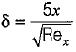QUESTION: 3

### The velocity profile for turbulent layer over a flat plate is

Solution:

Turbulent velocity profile follows 1/7 power law, hence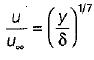will be velocity distribution.

QUESTION: 4

The turbulent boundary layer thickness varies as

Solution:

For turbulent flow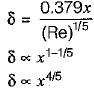QUESTION: 5

According to Blasius, the local skin friction coefficient in the boundary layer over a flat plate is given by

Solution:

Local skin friction coefficient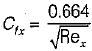QUESTION: 6

The thickness of laminar boundary layer at a distance 'x' from the leading edge over a flat plate varies as

Solution: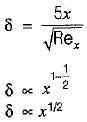QUESTION: 7

The Von-karman momentum integral equation expressed as (where θ is momentum thickness)

Solution:

Von-karman momentum integral equation is expressed as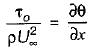QUESTION: 8

Consider the following statements:
Boundary layer separation is caused by:
1. An adverse pressure gradient
2. Sudden entrapping of air
3. Reduction of pressure gradient to zero
4. Release of bubbles from the fluid when the pressure goes below the vapor pressure

Q. Which of the above statements is/are correct?

Solution:
QUESTION: 9

Air flows past a golf bail of 20 mm radius. It is observed that the boundary layer becomes turbulent at Reynolds number of 2 x 105. If the kinematic viscosity of air is 1.5 x 10-5 m2/sec then the speed of air when the flow becomes turbulent is

Solution: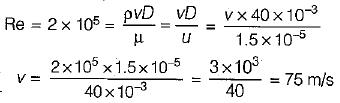QUESTION: 10

Blasius equation for a flat plate laminar boundary layer flow is a third order​

Solution: